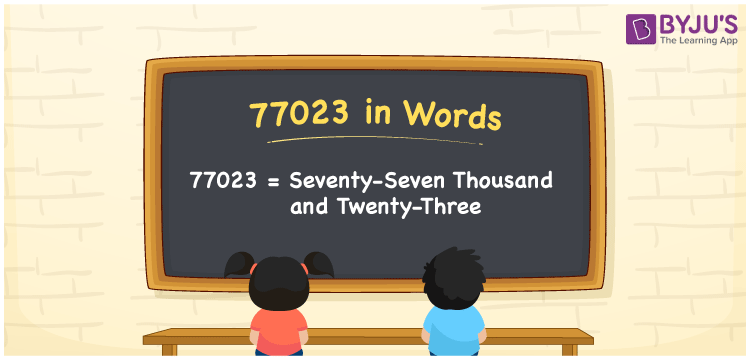# 77023 in Words

77023 in words is expressed as “seventy-seven thousand and twenty-three”. Learning the number names is essential for everyone as it is used in our daily life activities. For example, in a cheque, 77023 is written as Seventy-seven thousand and twenty-three only.Also, the number 77023 is a cardinal number. In this article, we are going to learn how to write the number 77023 in words using the place value system.

 77023 in Words: Seventy-seven Thousand and Twenty-three. Seventy-seven Thousand and Twenty-three in Numerical Form: 77023.

## 77023 in English Words## How to Write 77023 in Words?

The place value table for 77023 is given below:

 Ten-thousands Thousands Hundreds Tens Ones 7 7 0 2 3

The expanded form of 77023 is as follows:

= 7 × Ten thousand + 7 × Thousand + 0 × Hundred + 2 × Ten + 3 × One

= 7 × 10000 + 7 × 1000 + 0 × 100 + 2 × 10 + 3 × 1

= 70000 + 7000 + 20 + 3

= 77023

= Seventy-seven thousand and twenty-three

Hence, 77023 in words is seventy-seven thousand and twenty-three.

77023 in words – Seventy-seven thousand and twenty-three

Is 77023 an odd number? – Yes

Is 77023 an even number? – No

Is 77023 a perfect square number? – No

Is 77023 a perfect cube number? – No

Is 77023 a prime number? – Yes

Is 77023 a composite number? – No

## Frequently Asked Questions on 77023 in Words

Q1

### How to spell 77023?

77023 in words is seventy-seven thousand and twenty-three.

Q2

### Simplify 77000 + 23, and express it in words.

Simplifying 77000 + 23, we get 77023. Hence, 77023 in words is seventy-seven thousand and twenty-three.

Q3

### Is 77023 a prime number?

Yes, 77023 is a prime number.